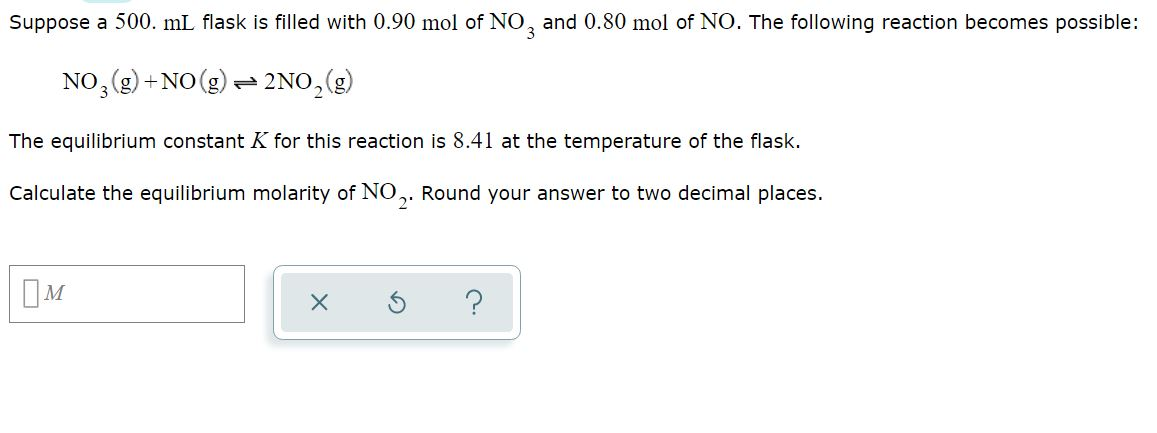# Suppose a 500. ml flask is filled with 0.90 mol of NO, and 0.80 mol of...

###### Question:Suppose a 500. ml flask is filled with 0.90 mol of NO, and 0.80 mol of NO. The following reaction becomes possible: NO2(g) + NO(g) + 2NO, (g) The equilibrium constant K for this reaction is 8.41 at the temperature of the flask. Calculate the equilibrium molarity of NO. Round your answer to two decimal places. IM x 3 ?